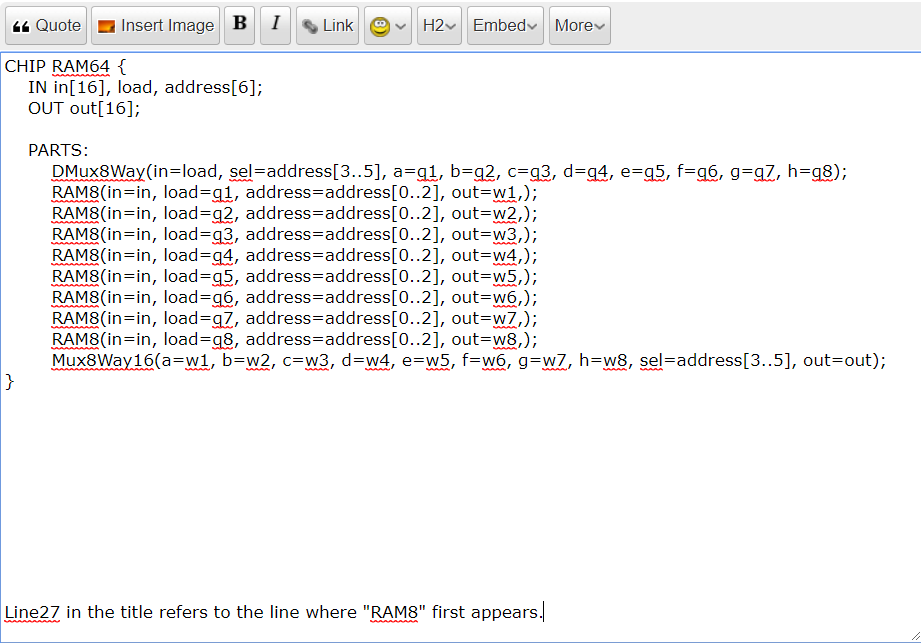# RAM64 Line27, a pin name is expectedClassicListThreaded7 messagesOpen this post in threaded view
|

## RAM64 Line27, a pin name is expected

Open this post in threaded view
|

## Re: RAM64 Line27, a pin name is expected

Open this post in threaded view
|

## Re: RAM64 Line27, a pin name is expected

 Fine
Open this post in threaded view
|

## Re: RAM64 Line27, a pin name is expected

 Administrator Once you get it formatted and posted, I'll take a closer look and point out what I see.
Open this post in threaded view
|

## Re: RAM64 Line27, a pin name is expected

 CHIP RAM64 {     IN in, load, address;     OUT out;     PARTS:         DMux8Way(in=load, sel=address[3..5], a=q1, b=q2, c=q3, d=q4, e=q5, f=q6, g=q7, h=q8);         RAM8(in=in, load=q1, address=address[0..2], out=w1,);         RAM8(in=in, load=q2, address=address[0..2], out=w2,);         RAM8(in=in, load=q3, address=address[0..2], out=w3,);         RAM8(in=in, load=q4, address=address[0..2], out=w4,);         RAM8(in=in, load=q5, address=address[0..2], out=w5,);         RAM8(in=in, load=q6, address=address[0..2], out=w6,);         RAM8(in=in, load=q7, address=address[0..2], out=w7,);         RAM8(in=in, load=q8, address=address[0..2], out=w8,);         Mux8Way16(a=w1, b=w2, c=w3, d=w4, e=w5, f=w6, g=w7, h=w8, sel=address[3..5], out=out); } Line27 in the title refers to the line where "RAM8" first appears.